Courses

Capacitance and Electrostatic Potential Notes | Study Physics For JEE - Class 12

Class 12: Capacitance and Electrostatic Potential Notes | Study Physics For JEE - Class 12

The document Capacitance and Electrostatic Potential Notes | Study Physics For JEE - Class 12 is a part of the Class 12 Course Physics For JEE.
All you need of Class 12 at this link: Class 12

What is a Capacitor?

Capacitors are also known as Electric-condensers. A capacitor is a two-terminal electric component. It has the ability or capacity to store energy in the form of electric charge.

• The capacitor is an arrangement of two conductors generally carrying charges of equal magnitudes and opposite sign and separated by an insulating medium.
• A capacitor is a device that stores electric charge.
• Capacitors vary in shape and size, but the basic configuration is two conductors carrying equal but opposite charges.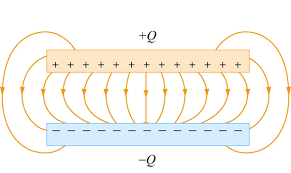What are Capacitors Used For?

• Capacitors have many important applications in electronics. Some examples include storing electric potential energy, delaying voltage changes when coupled with resistors, filtering out unwanted frequency signals, forming resonant circuits and making frequency-dependent and independent voltage dividers when combined with resistors.
• When charges are pulled apart, energy is associated with the pulling apart of charges, just like energy is involved in stretching a spring thus, some energy is stored in capacitors.
• In the uncharged state, the charge on either one of the conductors in the capacitor is zero. During the charging process a charge, Q is moved from one conductor to the other one, giving one conductor a charge, +Q and the other one a charge, -Q. A potential difference ΔV is created with the positively charged conductor at a higher potential than the negatively charged conductor. Note that whether charged or uncharged. the net charge on the capacitor as a whole is zero.

Note:

• The net charge on the capacitor as a whole is zero. When we say that a capacitor has a charge Q, we mean that the positively charged conductor has charge +Q and negatively charged conductor has a charge, -Q.
• In a circuit a capacitor is represented by the symbol: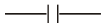What is Capacitance?

The capacitance of the conductor is defined as the charge required to increase the potential of a conductor by one unit. It is a scalar quantity. Unit of capacitance is farad in SI unis and its dimensional formula is M-1L-2I2T4

• Capacitance is nothing but the ability of a capacitor to store the energy in form of an electric charge. In other words, capacitance is the storing ability of a capacitor. It is measured in farads.
• 1 Farad: 1 Farad is the capacitance of a conductor for which 1-coulomb charge increases potential by 1 volt.
1 Farad =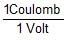1 µF = 10-6 F
1 nF = 10-9 F
or
1 pF = 10-12 F

Try yourself:What is the dimensional formula of capacitance of a capacitor?

Applications of Capacitors

1. Capacitors for Energy Storage

Since the late 18th century, capacitors are used to store electrical energy. Individual capacitors do not hold a great deal of energy, providing only enough power for electronic devices to use during temporary power outages or when they need additional power. There are many applications that use capacitors as energy sources and a few of them are as follows:

• Audio equipment
• Camera Flashes
• Power supplies
• Magnetic coils
• Lasers

Supercapacitors are capacitors that have high capacitances up to 2 kF. These capacitors store large amounts of energy and offer new technological possibilities in areas such as electric cars, regenerative braking in the automotive industry and industrial electrical motors, computer memory backup during power loss, and many others.

2. Capacitors for Power Conditioning

One of the important applications of capacitors is the conditioning of power supplies. Capacitors allow only AC signals to pass when they are charged blocking DC signals. This effect of a capacitor is majorly used in separating or decoupling different parts of electrical circuits to reduce noise, as a result of improving efficiency. Capacitors are also used in utility substations to counteract inductive loading introduced by transmission lines.

3. Capacitors as Sensors

Capacitors are used as sensors to measure a variety of things including humidity, mechanical strain, and fuel levels. Two aspects of capacitor construction are used in the sensing application – the distance between the parallel plates and the material between them. The former is used to detect mechanical changes such as acceleration and pressure and the latter is used in sensing air humidity.

4. Capacitors for Signal Processing

There are advanced applications of capacitors in information technology. Capacitors are used by Dynamic Random Access Memory (DRAM) devices to represent binary information as bits. Capacitors are also used in conjunction with inductors to tune circuits to particular frequencies, an effect exploited by radio receivers, speakers, and analog equalizers.

Factors Affecting Capacitance

1. Dielectric
The effect of dielectric on capacitance is that the greater the permittivity of the dielectric the greater the capacitance, likewise lesser the permittivity of the dielectric the lesser is the capacitance. Some materials offer less opposition to the field flux for a given amount of field force. Materials with greater permittivity allow more field flux, hence greater charge is collected.
2. Plate Spacing
The effect of spacing on the capacitance is that it is inversely proportional to the distance between the plates. Mathematically it is given as: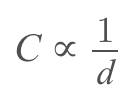3. Area of the Plates
The effect of the area of the plate is that the capacitance is directly proportional to the area. The larger the plate area more is the capacitance value. Mathematically it is given as C∝A

Capacitance of an Isolated Conductor

When a conductor is charged its potential increases. It is found that for an isolated conductor (conductor should be of finite dimension so that potential of infinity can be assumed to be zero) potential of the conductor is proportional to the charge given to it.
q = charge on conductor
V = potential of conductor

⇒ q = CV

Where C is proportionally constant called capacitance of the conductor.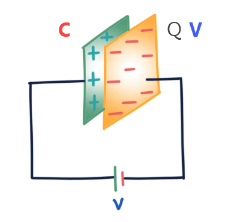Capacitance of an isolated conductor depends on the following factors

1. Shape and size of the conductor
On increasing the size, capacitance increase.
2. On surrounding medium
With the increase in dielectric constant K, capacitance increases.
3. Presence of other conductors
When a neutral conductor is placed near a charged conductor capacitance of conductors increases.

Capacitance of a conductor does not depend on

1. Charge on the conductor
2. Potential of the conductor
3. The potential energy of the conductor

Ex.1 Find out the capacitance of an isolated spherical conductor of radius R.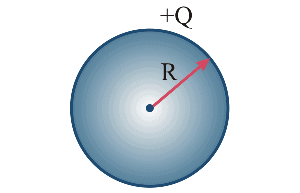Let there is charge Q on the sphere.
Therefore, Potential V = KQ/R
Hence by the formula: Q = CV

Q = CKQ/R
C = 4πε0R

• If the medium around the conductor is vacuum or air
Cvacuum = 4πε0R
R = Radius of the spherical conductor. (maybe solid or hollow)
• If the medium around the conductor is a dielectric of constant K from the surface of a sphere to infinity then
Cmedium = 4πε0KR
•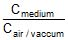= K = dielectric constant

What is Electrostatic Potential?

The electrostatic potential is also known as the electric field potential, electric potential, or potential drop is defined as:

The amount of work that is done in order to move a unit charge from a reference point to a specific point inside the field without producing an acceleration.

• Electric potential is a scalar property of every point in the region of the electric field. At a point in the electric field, the potential is defined as the interaction energy of a unit positive charge. If at a point in the electric field a charge q0 has potential energy U. then the electric potential at that point can be given as: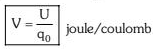• The potential energy of a charge in an electric field is defined as the work done in bringing the charge from infinity to the given point in the electric field. Similarly, we can define electric potential as work done in bringing a unit positive charge from infinity to the given point against the electric forces.
• Potential at a point can be physically interpreted as the work done within the conservative field in displacing a unit (+ve) charge from infinity to that point.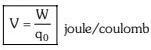Potential Energy and Potential Difference

• Electric Potential is the ‘push’ of electricity through a circuit. It’s easy to confuse electric potential with electric current, so it helps to think of electric current as of the water in our shower and electric potential as the water pressure. Like water pressure, the varying voltage can increase or decrease the flow of electricity.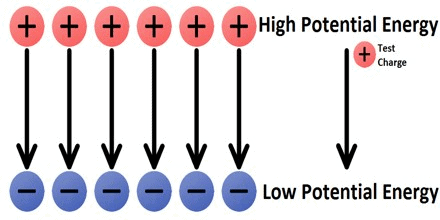Test charge moves from high potential to low potential

• Electric Potential is a scalar quantity denoted by V, equal to the electric potential energy of any charged particle at any location (measured in joules) divided by the charge of that particle (measured in coulombs). By dividing out the charge on the particle a remainder is obtained that is a property of the electric field itself.
• This value can be calculated in either a static (time-invariant) or a dynamic (varying with time) electric field at a specific time in units of joules per coulomb, or volts (V). The electric potential at infinity is assumed to be zero. The concept of electric potential is useful in understanding electrical phenomena; only differences in potential energy are measurable.
• If an electric field is defined as the force per unit charge, then by analogy an electric potential can be thought of as the potential energy per unit charge. Therefore, the work done in moving a unit charge from one point to another (e.g., within an electric circuit) is equal to the difference in potential energies at each point. In the International System of Units (SI), an electric potential is expressed in units of joules per coulomb (i.e., volts), and differences in potential energy are measured with a voltmeter.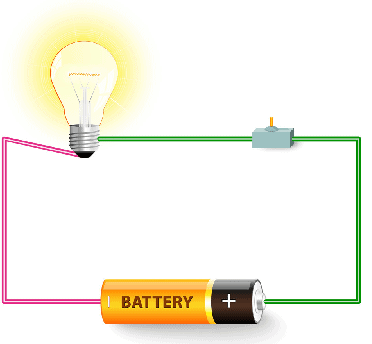Simple electric circuit

Electric Potential in Circuits

• Charge moving through the wires of the circuit will encounter changes in electric potential as it traverses the circuit. Within the electrochemical cells of the battery, there is an electric field established between the two terminals, directed from the positive terminal towards the negative terminal.
• As such, the movement of a positive test charge through the cells from the negative terminal to the positive terminal would require work, thus increasing the potential energy of every Coulomb of charge that moves along this path.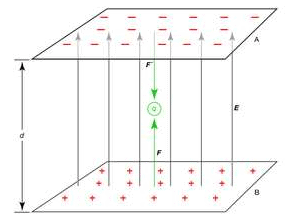Electric potential movement in circuits
• This corresponds to a movement of the positive charge against the electric field. It is for this reason that the positive terminal is described as the high potential terminal. The charge would lose potential energy as moves through the external circuit from the positive terminal to the negative terminal.
• The negative terminal is described as the low potential terminal. This assignment of high and low potential to the terminals of an electrochemical cell presumes the traditional convention that electric fields are based on the direction of movement of positive test charges.
• Chemical energy is transformed into electric potential energy within the internal circuit (i.e., the battery). Once at the high potential terminal, a positive test charge will then move through the external circuit and do work upon the light bulb or the motor or the heater coils, transforming its electric potential energy into useful forms for which the circuit was designed.
• The positive test charge returns to the negative terminal at low energy and low potential, ready to repeat the cycle (or should we say circuit) all over again.

Electric Potential Difference

• Electric potential energy is defined as the total amount of work done by an external agent in moving a charge from an arbitrarily chosen reference point, which we usually take as infinity, as it is assumed that the electrical potential of a charge at infinity is zero. To that position without any acceleration. For any charge, an electric potential is obtained by dividing the electric potential energy by the quantity of charge.
• In an electrical circuit, the electric potential between two points is defined as the amount of work done by an external agent in moving a unit charge from one point to another.

Mathematically,

E = W/Q

Where,
= electrical potential difference between two points
= Work done in moving a charge from one point to another
Q = the quantity of charge in coulombs
The potential difference is measured by an instrument called a voltmeter. The two terminals of a voltmeter are always connected parallel across the points whose potential is to be measured.

Try yourself: If a charged body is moved in an electric field against the Coulomb force, then ________

The document Capacitance and Electrostatic Potential Notes | Study Physics For JEE - Class 12 is a part of the Class 12 Course Physics For JEE.
All you need of Class 12 at this link: Class 12Use Code STAYHOME200 and get INR 200 additional OFF Use Coupon Code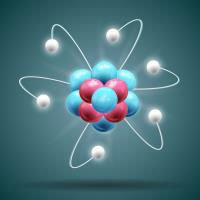Physics For JEE

187 videos|516 docs|256 tests

Track your progress, build streaks, highlight & save important lessons and more!

,

,

,

,

,

,

,

,

,

,

,

,

,

,

,

,

,

,

,

,

,

;